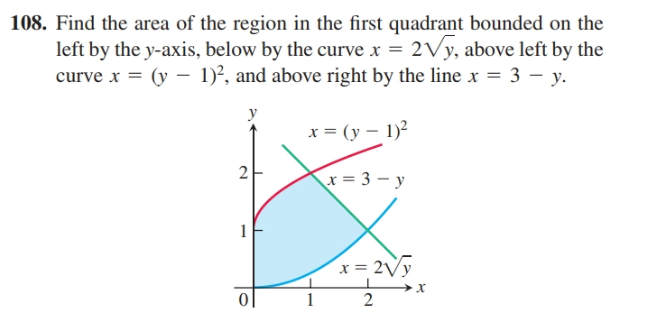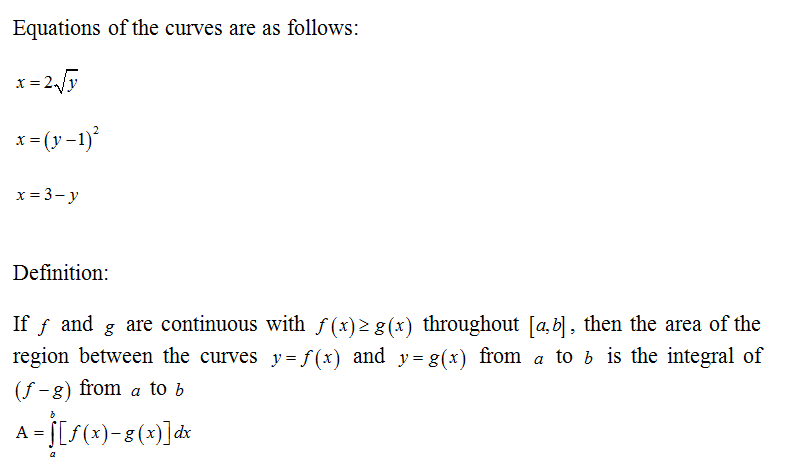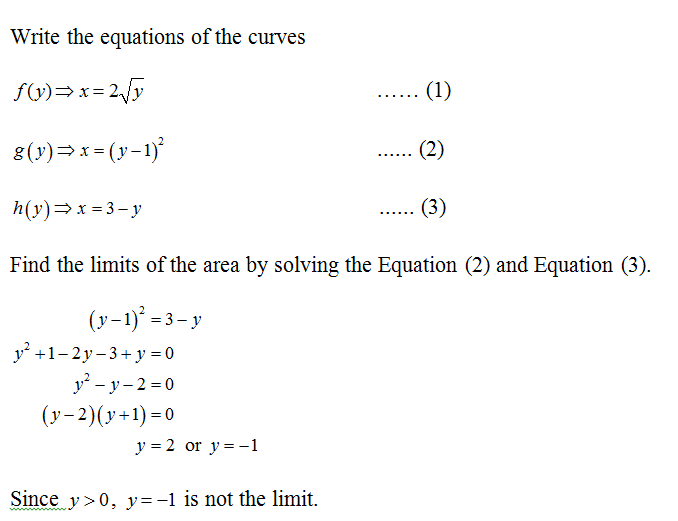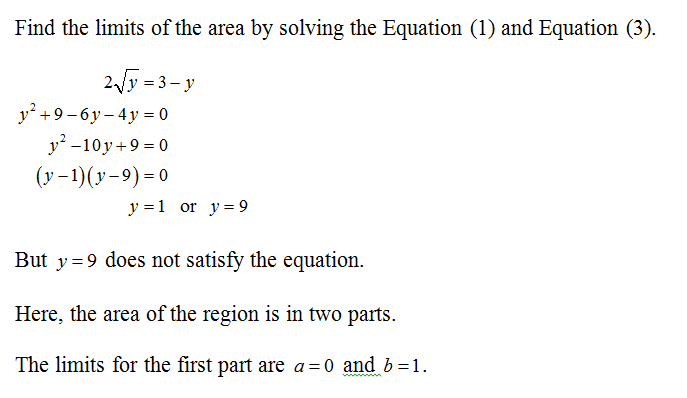# 108. Find the area of the region in the first quadrant bounded on theleft by the y-axis, below by the curve x = 2Vy, above left by thecurve x = (y – 1)², and above right by the line x = 3 – y.yx = (y – 1)?2x = 3 – y1x = 2Vy0|2

Question
2 viewshelp_outlineImage Transcriptionclose108. Find the area of the region in the first quadrant bounded on the left by the y-axis, below by the curve x = 2Vy, above left by the curve x = (y – 1)², and above right by the line x = 3 – y. y x = (y – 1)? 2 x = 3 – y 1 x = 2Vy 0| 2 fullscreen
check_circle

Given informationCalculation:Step 3...

### Want to see the full answer?

See Solution

#### Want to see this answer and more?

Solutions are written by subject experts who are available 24/7. Questions are typically answered within 1 hour.*

See Solution
*Response times may vary by subject and question.
Tagged in